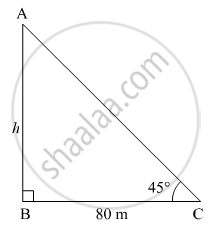Share

# A Person is Standing at a Distance of 80 M from a Church Looking at Its Top. the Angle of Elevation is of 45°. Find the Height of the Church. - Geometry

ConceptHeights and Distances

#### Question

A person is standing at a distance of 80 m from a church looking at its top. The angle of elevation is of 45°. Find the height of the church.

#### Solution

Let AB be the church and C be the position of the person from the church.
Suppose the height of the church be h m.In right ∆ABC,
$\tan45^\circ = \frac{AB}{BC}$
$\Rightarrow 1 = \frac{h}{80}$
$\Rightarrow h = 80$  m

Thus, the height of the church is 80 m.

Is there an error in this question or solution?

#### APPEARS IN

Balbharati Solution for Balbharati Class 10 Mathematics 2 Geometry (2018 to Current)
Chapter 6: Trigonometry
Practice set 6.2 | Q: 1 | Page no. 137

#### Video TutorialsVIEW ALL 

Solution A Person is Standing at a Distance of 80 M from a Church Looking at Its Top. the Angle of Elevation is of 45°. Find the Height of the Church. Concept: Heights and Distances.
S